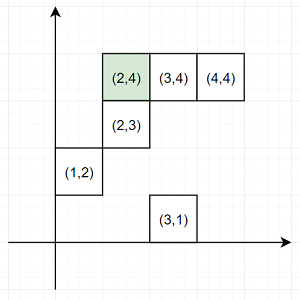# Program to find nearest point that has the same x or y coordinate using Python

Suppose we have a set of points given in an array called pts. We also have another point (x, y) which is our current location. We are defining a valid point as, a point which shares the same x-coordinate or the same y-coordinate as our current point. We have to return the index of the valid point with the smallest Manhattan distance from our current location (x, y). If there are more than one points, then return the valid point with the smallest index. (Note: the Manhattan distance between two points (a, b) and (p, q) is |a - p| + |b - q|.

So, if the input is like pts = [(1,2),(3,1),(3,4),(2,3),(4,4)] pt = (2,4), then the output will be 2 as there are two nearest points (3,4) and (2,3), but the index of (3,4) is smaller.To solve this, we will follow these steps −

• x, y := pt

• idx := -1

• smallest := infinity

• for each p in pts, do

• if p is same as x or p is same as y, then

• dist := |x - p| + |y - p|

• if dist < smallest, then

• idx := index of p in pts

• smallest := dist

• otherwise when dist is same as smallest, then

• if index of p in pts < idx, then

• idx := index of p in pts

• smallest := dist

• return idx

Let us see the following implementation to get better understanding −

## Example

Live Demo

def solve(pts, pt):
x, y = pt
idx = -1
smallest = float("inf")
for p in pts:
if p == x or p == y:
dist = abs(x - p) + abs(y - p)
if dist < smallest:
idx = pts.index(p)
smallest = dist
elif dist == smallest:
if pts.index(p) < idx:
idx = pts.index(p)
smallest = dist
return idx
pts = [(1,2),(3,1),(3,4),(2,3),(4,4)]
pt = (2,4)
print(solve(pts, pt))

## Input

[(1,2),(3,1),(3,4),(2,3),(4,4)], (2,4)

## Output

2